Homework Help Question & Answers

# A pulley has a rotational inertia i=0.0030kgm^2 and radius r=0.050m . A 0.6kg block hanging from...

A pulley has a rotational inertia i=0.0030kgm^2 and radius r=0.050m . A 0.6kg block hanging from a massless rope hanging over the pulley descends as the cord unwinds without slipping, the magnitude of the blocks acceleration is?

#### Homework Answers

Answer #1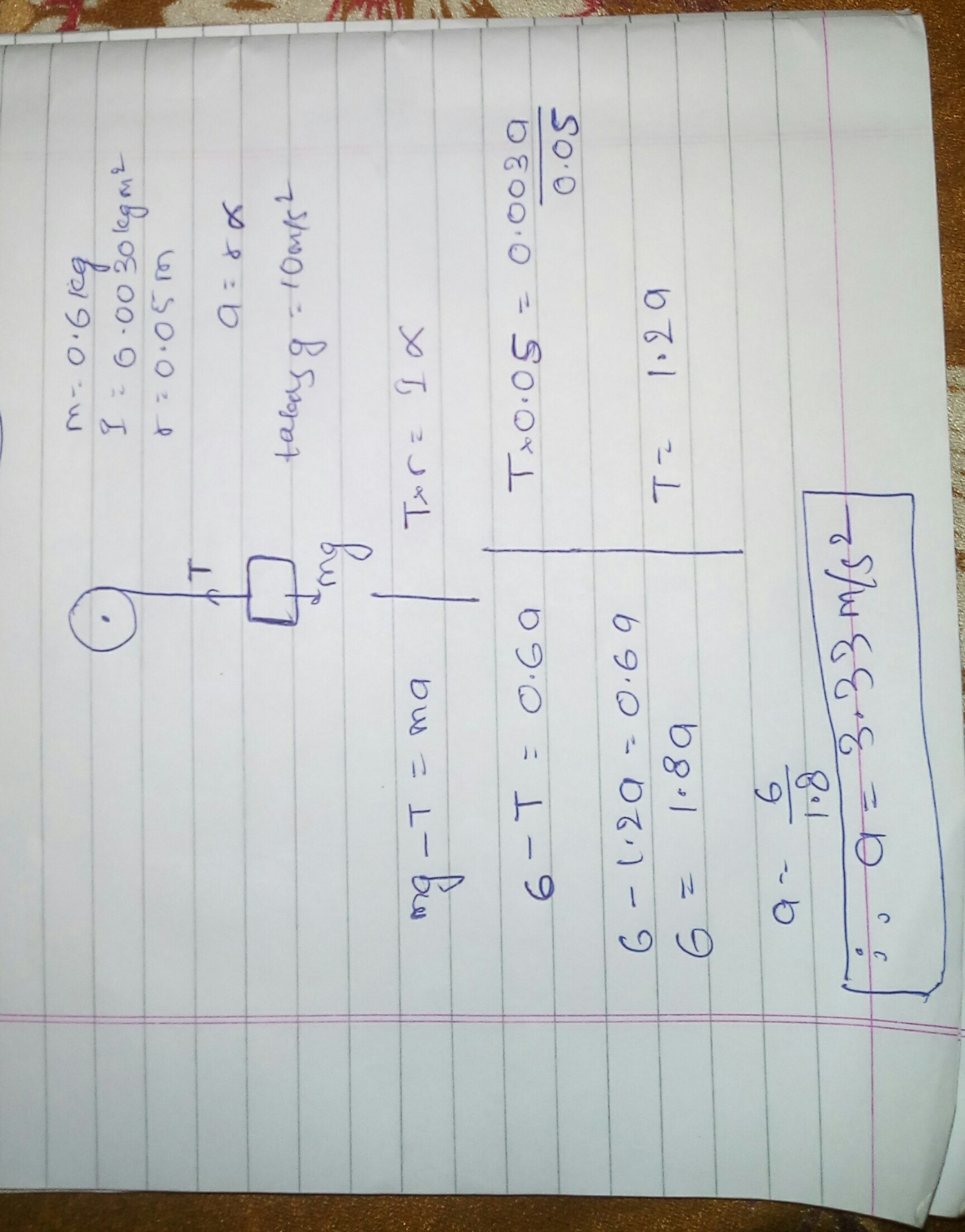a=3.33m/s2

Know the answer?
Your Answer:

#### Post as a guest

Your Name:

What's your source?

#### Earn Coin

Coins can be redeemed for fabulous gifts.

Not the answer you're looking for? Ask your own homework help question. Our experts will answer your question WITHIN MINUTES for Free.
Similar Homework Help Questions
• ### MR A pulley of mass 3M and radius R is mounted on ftictionless bearings and supported...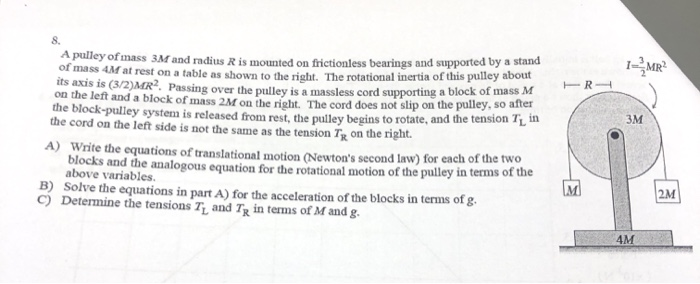MR A pulley of mass 3M and radius R is mounted on ftictionless bearings and supported by a stand of mass 4M at rest on a table as shown to the right. The rotational inertia of this pulley about its axis is (3/2)MR2. Passing over the pulley is a massless cord supporting a block of mass M on the left and a block of mass 2M on the right. The cord does not slip on the pulley, so after the...

• ### In the figure, block 1 has mass m1 = 440 g, block 2 has mass m2...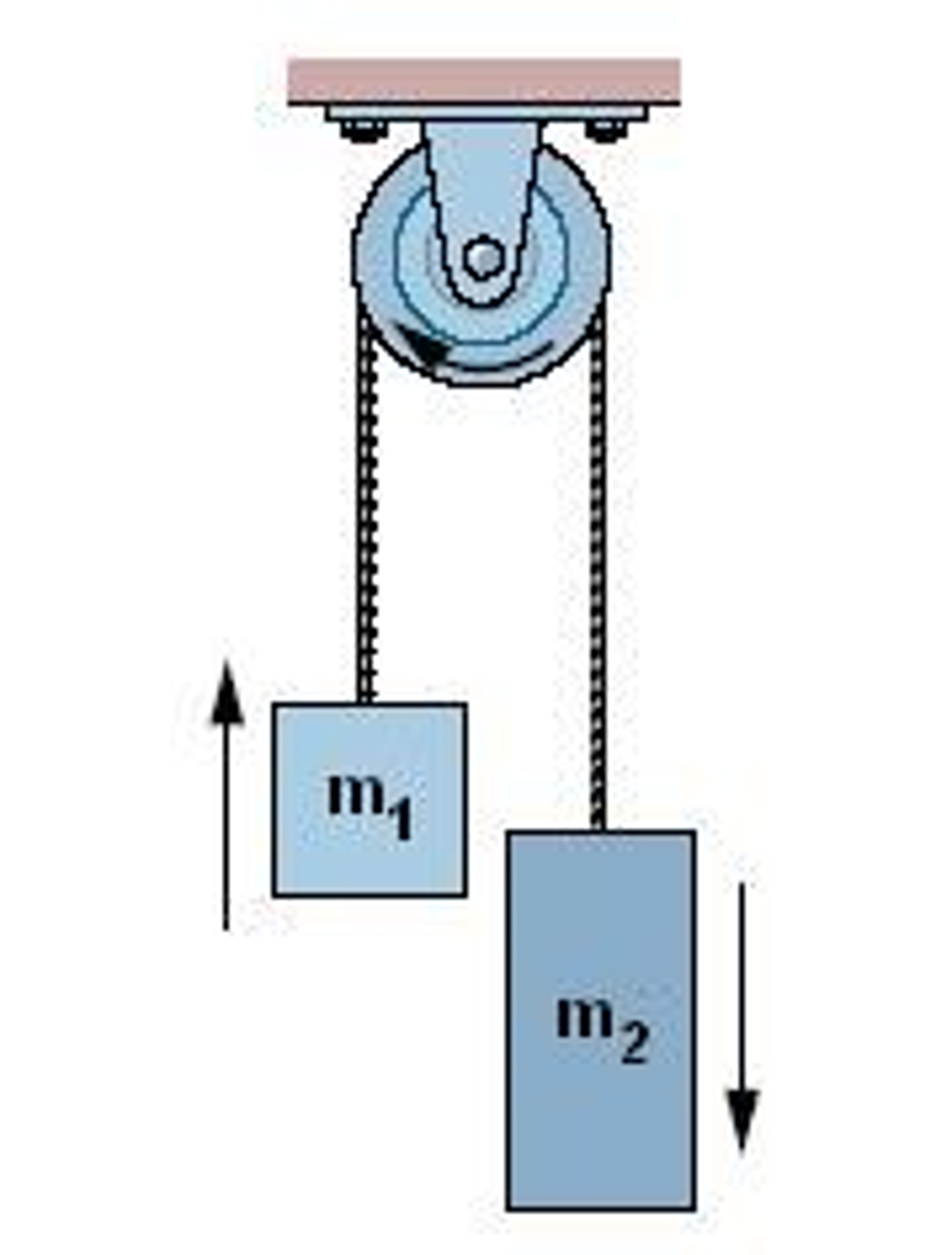In the figure, block 1 has mass m1 = 440 g, block 2 has mass m2 = 580 g, and the pulley is on a frictionless horizontal axle and has radius R = 5.2 cm. When released from rest, block 2 falls 75 cm in 4.9 s without the cord slipping on the pulley. (a) What is the magnitude of the acceleration of the blocks? What are (b) tension T2 (the tension force on the block 2) and (c) tension...

• ### In the figure, block 1 has mass m1 = 460 g, block 2 has mass m2...In the figure, block 1 has mass m1 = 460 g, block 2 has mass m2 = 592 g, and the pulley is on a frictionless horizontal axle and has radius R = 4.70 cm. When released from rest, block 2 falls 74.7 cm in 5.32 s without the cord slipping on the pulley. (a) What is the magnitude of the acceleration of the blocks? What are (b) tension T2 (the tension force on the block 2) and (c) tension...

• ### The pulley in the figure (Figure 1) has radius R and a moment of inertia I. The rope does not slip...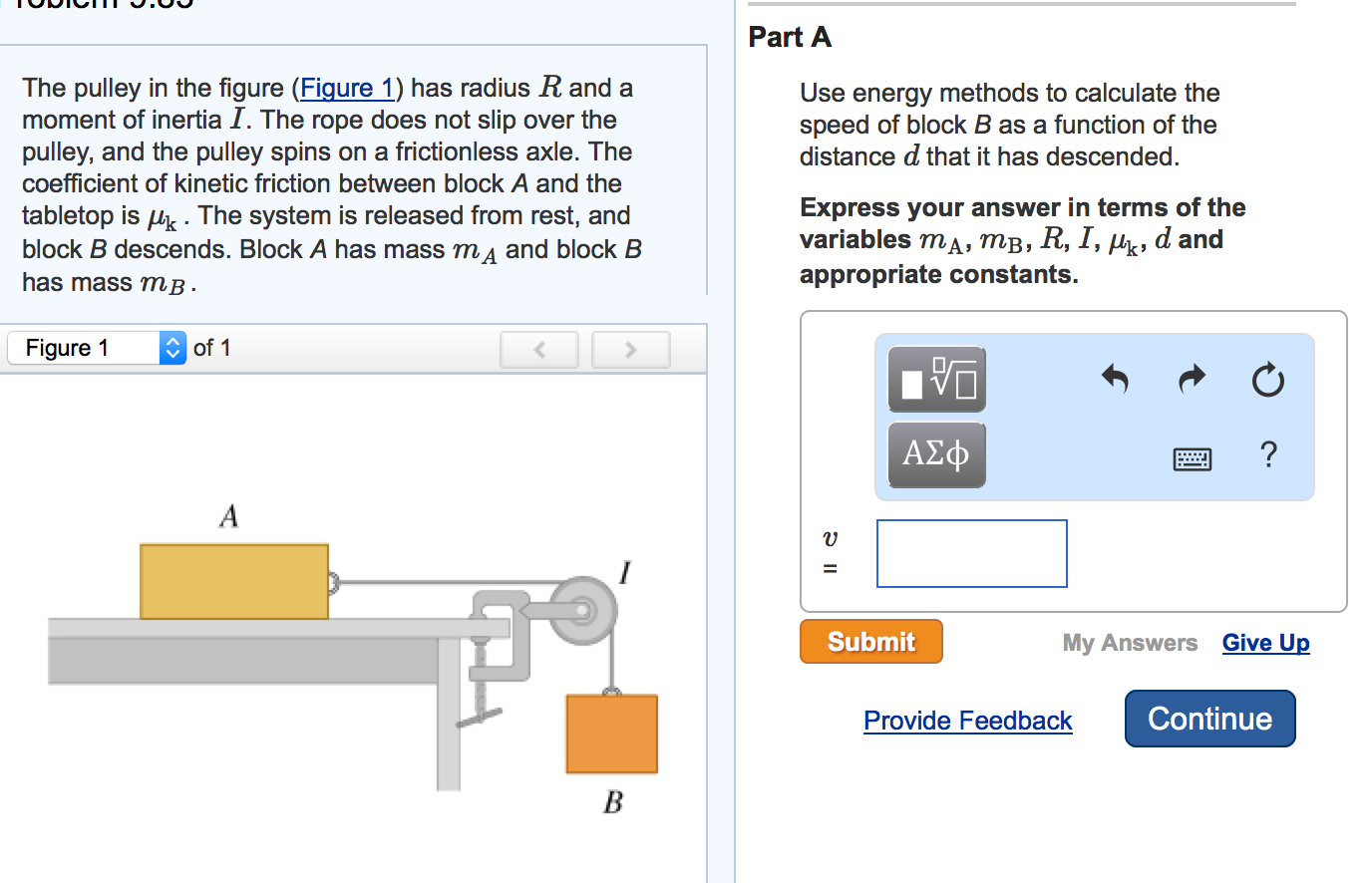The pulley in the figure (Figure 1) has radius R and a moment of inertia I. The rope does not slip over the pulley, and the pulley spins on a frictionless axle. The coefficient of kinetic friction between block A and the tabletop is mu_k . The system is released from rest, and block B descends. Block A has mass m_A and block B has mass m_B Use energy methods to calculate the speed of block B as a function of the distance d that it has descended. Express your answer in terms of the variables m_A, m_B, R, I, mu_k, d and appropriate constants.

• ### 3 (15 points) The pulley in the figure has radius R and a moment of inertia I. The rope does not slip over the pulley,...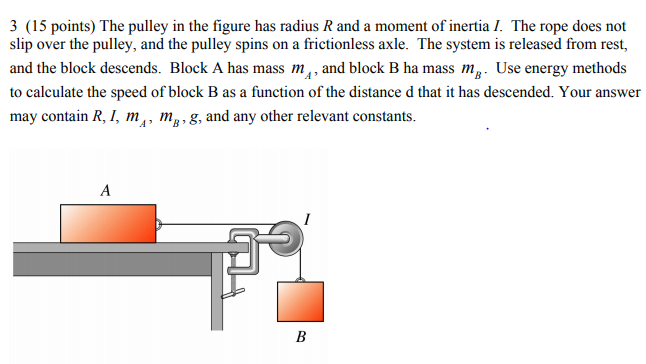3 (15 points) The pulley in the figure has radius R and a moment of inertia I. The rope does not slip over the pulley, and the pulley spins on a frictionless axle. The system is released from rest, and the block descends. Block A has mass my, and block B ha mass mg. Use energy methods to calculate the speed of block B as a function of the distance d that it has descended. Your answer may contain R,...

• ### A block of mass m is hanging from a cord that is wrapped around a pulley...

A block of mass m is hanging from a cord that is wrapped around a pulley with radius R and moment of inertia I. When the block is released from rest, the pulley will rotate counterclockwise. Part A Solve for the acceleration of the block (your answer can include m,g,R, and I) Part B Draw a free body diagram showing the forces that act on the block Part C What happens to the acceleration of the block if the moment...

• ### 3. In the figure above, a spool or pulley with moment of inertia MR2 is hanging...

3. In the figure above, a spool or pulley with moment of inertia MR2 is hanging from a ceiling by a (massless, unstretchable) string that is wrapped around it at a radius R, while a block of equal mass M is hung on a second string that is wrapped around it at a radius r as shown. Find the magnitude of the acceleration of the the central pulley.

• ### In the figure, block 1 has mass m - 490 g, block 2 has mass m2...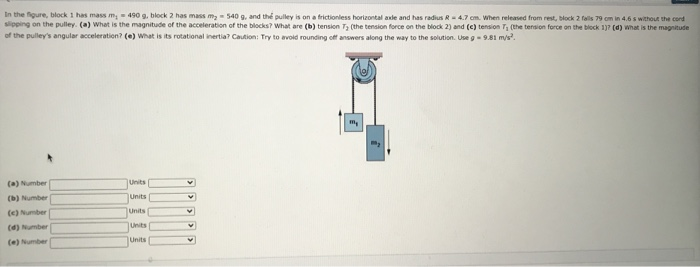In the figure, block 1 has mass m - 490 g, block 2 has mass m2 - 5409, and the pulley is on a frictionless horizontal and and has radius R - 4.7 cm. When released from rest, block 2 fois 79 cm in 4.6 s without the cord slipping on the pulley (a) What is the magnitude of the acceleration of the blocks? What are (b) tension Tz (the tension force on the block 2) and (c) tension T,...

• ### In the figure, block 1 has mass mi = 430 g, block 2 has mass m2...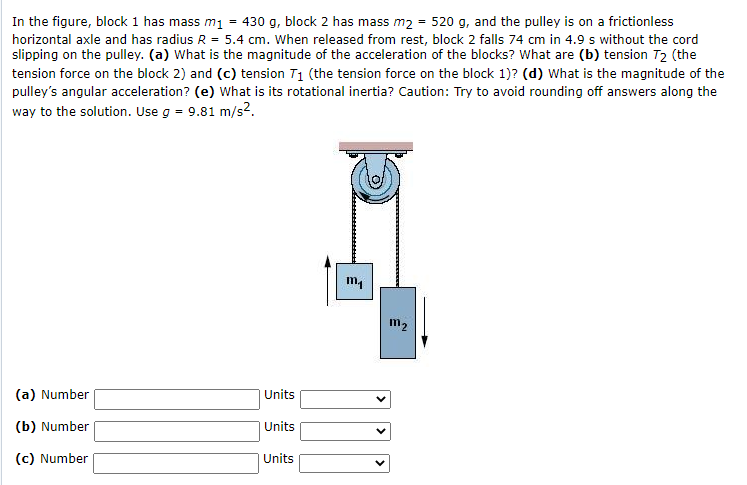In the figure, block 1 has mass mi = 430 g, block 2 has mass m2 = 520 g, and the pulley is on a frictionless horizontal axle and has radius R = 5.4 cm. When released from rest, block 2 falls 74 cm in 4.9 s without the cord slipping on the pulley. (a) What is the magnitude of the acceleration of the blocks? What are (b) tension 72 (the tension force on the block 2) and (c) tension...

• ### Rotational Motion Around a Pulley

Two identical 1.0-kg blocks are connected by a massless string over a frictionless pulley of radius R = 6.000 cm and rotational inertia I = 0.003 kg m2 (see Figure).The string does not slip on the pulley, so the horizontal tension is different than the vertical tension. What is the magnitude of the angular acceleration of thepulley?